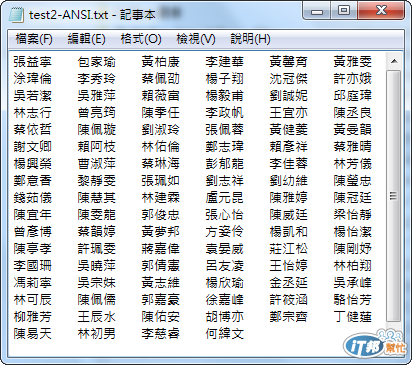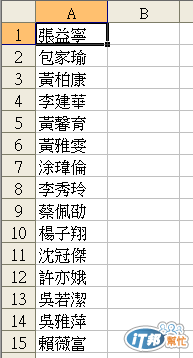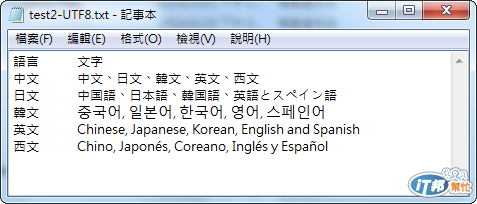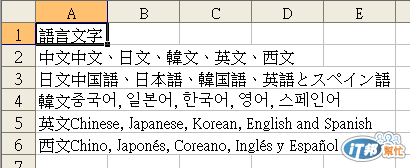#DAY 18
1

## Excel VBA 的眉眉角角Day18: 讀取UTF8檔案後，利用Split功能切割以及Resize與Application.Transpose的介紹

``````Sub Day18_ANSI()
Dim strPath As String
Dim strFileType As String
Dim strFile As String

'選取要轉入的檔案
strPath = SpecialFolders("MyDocuments")
strFileType = "*.txt"
strFile = SelectFile(strPath, strFileType)
If Len(strFile) = 0 Then Exit Sub

'以二進位方式讀入資料，在轉成文字字串
Dim b() As Byte
Open strFile For Binary As #1
ReDim b(LOF(1) - 1)
Get #1, , b
Close
b = StrConv(b, vbUnicode)

'依照Tab字元切割資料，由A欄最後一行空白開始匯入
iNewRow = Range("A65535").End(xlUp).Row + 1
If Range("A1") = "" And iNewRow = 2 Then iNewRow = 1

tmp = Split(b, vbTab)
For i = 0 To UBound(tmp)
Debug.Print tmp(i)
Range("A" & iNewRow).Offset(i, 0) = tmp(i)
Close
Next
End Sub
````````````Public Function Read_UTF8_Text_File(strPath As String)
'讀取UTF8純文字檔

End Function
``````

``````Sub Day18_UTF8()
Dim strPath As String
Dim strFileType As String
Dim strFile As String

'選取要轉入的檔案
strPath = SpecialFolders("MyDocuments")
strFileType = "*.txt"
strFile = SelectFile(strPath, strFileType)
If Len(strFile) = 0 Then Exit Sub

iNewRow = Range("A65535").End(xlUp).Row + 1
If Range("A1") = "" And iNewRow = 2 Then iNewRow = 1

'由A欄第一個空白行輸入資料，透過Resize與 Application.Transpose方式塞入切割後的陣列資料
Range("A" & iNewRow).Resize(UBound(var_String) - LBound(var_String) + 1).Value = Application.Transpose(var_String)
End Sub
``````Excel VBA 的眉眉角角30